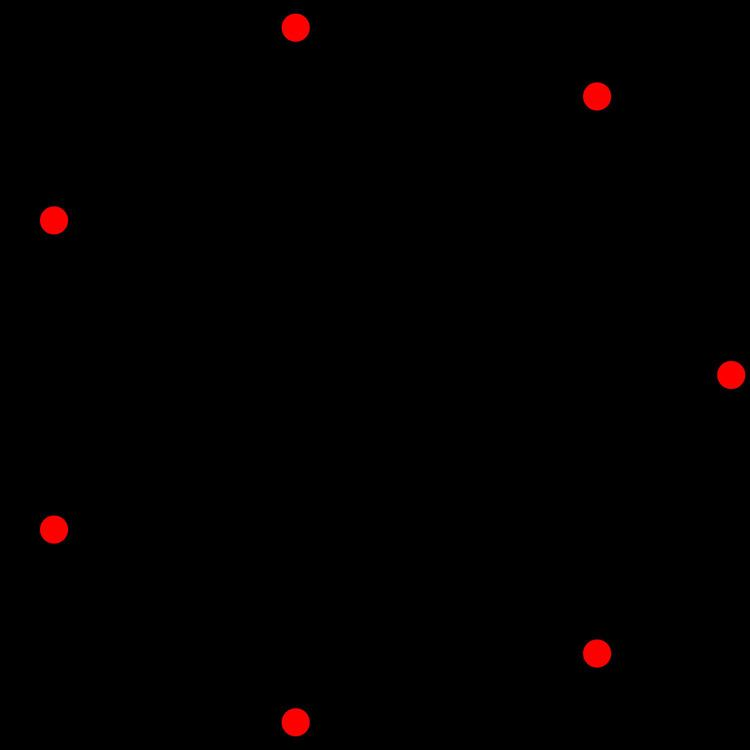# 6 polytope

Updated on
Covid-19In six-dimensional geometry, a six-dimensional polytope or 6-polytope is a polytope, bounded by 5-polytope facets.

## Definition

A 6-polytope is a closed six-dimensional figure with vertices, edges, faces, cells (3-faces), 4-faces, and 5-faces. A vertex is a point where six or more edges meet. An edge is a line segment where four or more faces meet, and a face is a polygon where three or more cells meet. A cell is a polyhedron. A 4-face is a polychoron, and a 5-face is a 5-polytope. Furthermore, the following requirements must be met:

• Each 4-face must join exactly two 5-faces (facets).
• Adjacent facets are not in the same five-dimensional hyperplane.
• The figure is not a compound of other figures which meet the requirements.
• ## Characteristics

The topology of any given 6-polytope is defined by its Betti numbers and torsion coefficients.

The value of the Euler characteristic used to characterise polyhedra does not generalize usefully to higher dimensions, and is zero for all 6-polytopes, whatever their underlying topology. This inadequacy of the Euler characteristic to reliably distinguish between different topologies in higher dimensions led to the discovery of the more sophisticated Betti numbers.

Similarly, the notion of orientability of a polyhedron is insufficient to characterise the surface twistings of toroidal polytopes, and this led to the use of torsion coefficients.

## Classification

6-polytopes may be classified by properties like "convexity" and "symmetry".

• A 6-polytope is convex if its boundary (including its 5-faces, 4-faces, cells, faces and edges) does not intersect itself and the line segment joining any two points of the 6-polytope is contained in the 6-polytope or its interior; otherwise, it is non-convex. Self-intersecting 6-polytope are also known as star 6-polytopes, from analogy with the star-like shapes of the non-convex Kepler-Poinsot polyhedra.
• A regular 6-polytope has all identical regular 5-polytope facets. All regular 6-polytope are convex.
• A semi-regular 6-polytope contains two or more types of regular 4-polytope facets. There is only one such figure, called 221.
• A uniform 6-polytope has a symmetry group under which all vertices are equivalent, and its facets are uniform 5-polytopes. The faces of a uniform polytope must be regular.
• A prismatic 6-polytope is constructed by the Cartesian product of two lower-dimensional polytopes. A prismatic 6-polytope is uniform if its factors are uniform. The 6-cube is prismatic (product of a squares and a cube), but is considered separately because it has symmetries other than those inherited from its factors.
• A 5-space tessellation is the division of five-dimensional Euclidean space into a regular grid of 5-polytope facets. Strictly speaking, tessellations are not 6-polytopes as they do not bound a "6D" volume, but we include them here for the sake of completeness because they are similar in many ways to 6-polytope. A uniform 5-space tessellation is one whose vertices are related by a space group and whose facets are uniform 5-polytopes.
• ## Regular 6-polytopes

Regular 6-polytopes can be generated from Coxeter groups represented by the Schläfli symbol {p,q,r,s,t} with t {p,q,r,s} 5-polytope facets around each cell.

There are only three such convex regular 6-polytopes:

• {3,3,3,3,3} - 6-simplex
• {4,3,3,3,3} - 6-cube
• {3,3,3,3,4} - 6-orthoplex
• There are no nonconvex regular polytopes of 5 or more dimensions.

For the 3 convex regular 6-polytopes, their elements are:

## Uniform 6-polytopes

Here are six simple uniform convex 6-polytopes, including the 6-orthoplex repeated with its alternate construction.

The expanded 6-simplex is the vertex figure of the uniform 6-simplex honeycomb, . The 6-demicube honeycomb, , vertex figure is a rectified 6-orthoplex and facets are the 6-orthoplex and 6-demicube. The uniform 222 honeycomb,, has 122 polytope is the vertex figure and 221 facets.

## References

Similar Topics
The Council of the Gods
Victoria Miro
Alan Fleischmann
Topics

 B i Link H2
 L